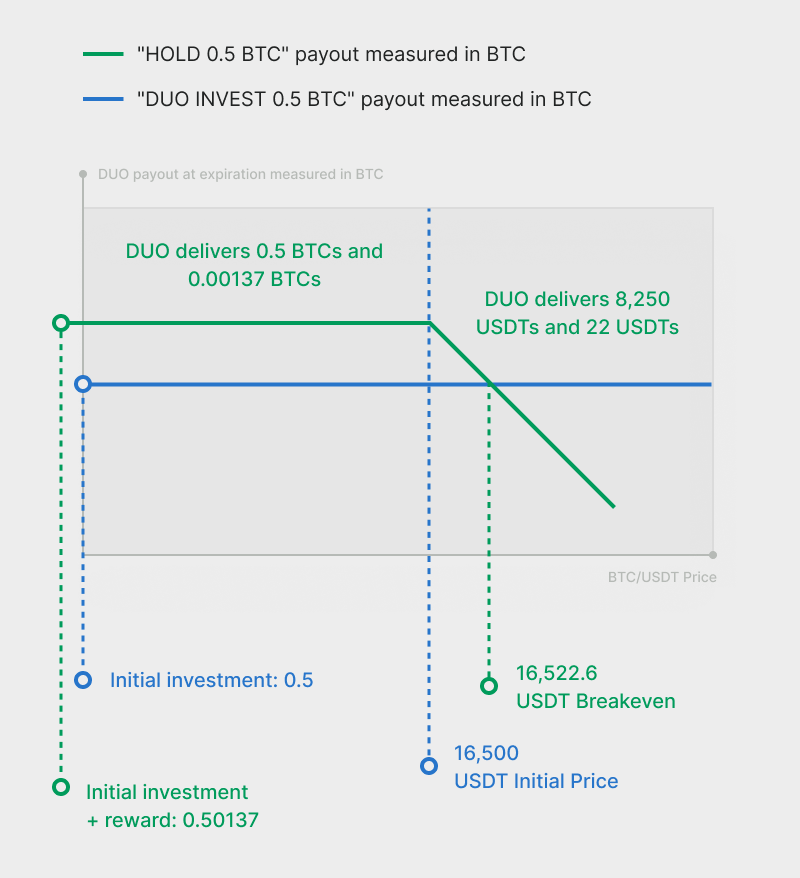< Back to category

# How does DUO work?

Depositing the invested digital currency into a DUO consists of swapping this currency’s potential volatility over the quote currency, for a fixed predetermined reward indicated by the Annual Percentage Rate (APR). The APR is scaled by the DUO’s timeframe (12 hours, 1 day or 2 days) and multiplied by the invested amount to calculate the exact reward amount paid to the user at expiration.

For example, using BTC as the invested digital currency, and USDT as the quote digital currency:

For 0.5 Bitcoin (BTC) invested in the BTC/USDT 12 hours DUO at 200% APR, the reward at expiration is: [0.5] * [12 / (365*24)] * [200%] = 0.00137 BTC.

There are two scenarios at expiration. If BTC doesn't exceed the initial price at the end of the term, you'll earn extra BTC. If BTC exceeds the initial price, BTCs will be sold at a price higher than the initial price and you'll receive USDTs.

#### Scenario one

If the price of BTC/USDT at expiration is lower than the initial price, you'll earn extra BTC.

The amount you'll receive by the expiration date is determined by the following formula: Invested amount × (1 + APR*(term in hours)/(24*365.0)) = 0.5*(1+ 200%*12 / (365*24)) = 0.50137 BTC

#### Scenario two

If the price of BTC/USDT at expiration is higher than, or equal to, the initial price, we'll sell BTC at the initial price and earn USDT.

The amount you'll receive by the expiration date is determined by the following formula: Invested amount × Initial price x (1 + APR*(term in hours)/(24*365.0)) = 0.5*16,500 * (1+ 200%*12 / (365*24)) = 8,272 USDTUltimately, whether you receive BTC or USDT back, you’ll want to ensure that these tokens have more value than what you initially deposited. The best way to achieve this is by seeking minimal volatility for the time the DUO is open.

The lower the pair’s volatility, the more likely the expiration price of BTC/USDT will stay near the initial price level.

## Didn’t find anything?

Our support will solve your issue as soon as possible

###### Wirex CommunityJoin Wirex Discord for the latest news, releases and updates